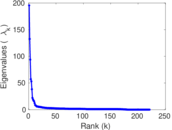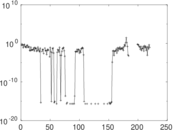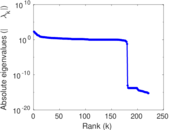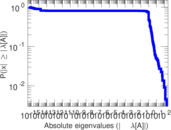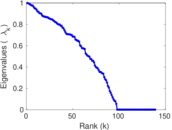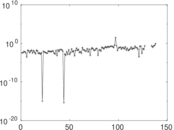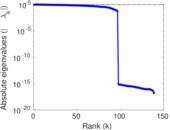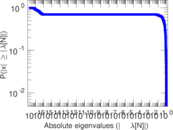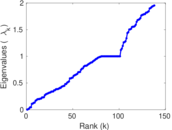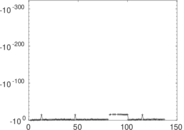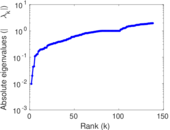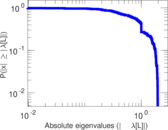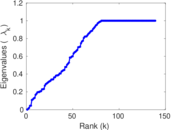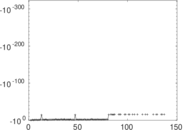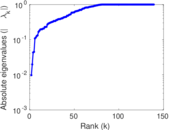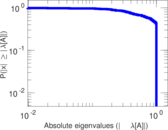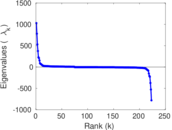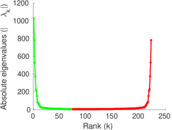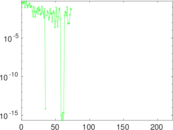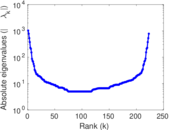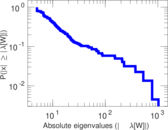# Wikiquote edits (mr)

This is the bipartite edit network of the Marathi Wikiquote. It contains users and pages from the Marathi Wikiquote, connected by edit events. Each edge represents an edit. The dataset includes the timestamp of each edit.

 Code `qmr` Internal name `edit-mrwikiquote` Name Wikiquote edits (mr) Data source http://dumps.wikimedia.org/ AvailabilityDataset is available for download Consistency checkDataset passed all tests Category Authorship network Dataset timestamp 2017-10-20 Node meaning User, article Edge meaning Edit Network formatBipartite, undirected Edge typeUnweighted, multiple edges Temporal dataEdges are annotated with timestamps

## Statistics

 Size n = 1,041 Left size n1 = 223 Right size n2 = 818 Volume m = 2,250 Unique edge count m̿ = 1,109 Wedge count s = 45,251 Claw count z = 2,333,956 Cross count x = 97,343,833 Square count q = 1,013 4-Tour count T4 = 191,370 Maximum degree dmax = 288 Maximum left degree d1max = 288 Maximum right degree d2max = 266 Average degree d = 4.322 77 Average left degree d1 = 10.089 7 Average right degree d2 = 2.750 61 Fill p = 0.006 079 58 Average edge multiplicity m̃ = 2.028 85 Size of LCC N = 799 Diameter δ = 17 50-Percentile effective diameter δ0.5 = 4.589 69 90-Percentile effective diameter δ0.9 = 7.926 85 Median distance δM = 5 Mean distance δm = 5.387 28 Gini coefficient G = 0.740 105 Balanced inequality ratio P = 0.202 222 Left balanced inequality ratio P1 = 0.162 667 Right balanced inequality ratio P2 = 0.262 222 Relative edge distribution entropy Her = 0.839 508 Power law exponent γ = 4.788 74 Tail power law exponent γt = 2.571 00 Tail power law exponent with p γ3 = 2.571 00 p-value p = 0.042 000 0 Left tail power law exponent with p γ3,1 = 2.091 00 Left p-value p1 = 0.752 000 Right tail power law exponent with p γ3,2 = 3.011 00 Right p-value p2 = 0.370 000 Degree assortativity ρ = −0.297 952 Degree assortativity p-value pρ = 3.583 93 × 10−24 Spectral norm α = 195.420 Algebraic connectivity a = 0.009 726 92 Spectral separation |λ1[A] / λ2[A]| = 1.474 88 Controllability C = 667 Relative controllability Cr = 0.649 464

## Plots

### Fruchterman–Reingold graph drawing### Degree distribution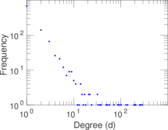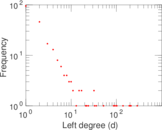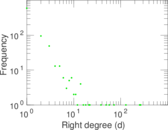### Cumulative degree distribution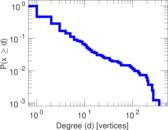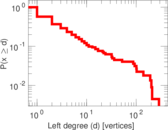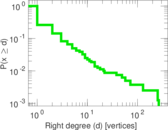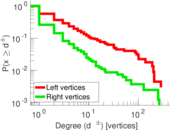### Lorenz curve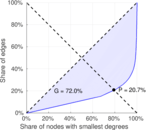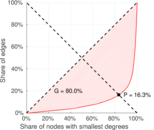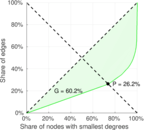### Spectral distribution of the adjacency matrix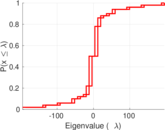### Spectral distribution of the normalized adjacency matrix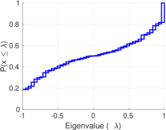### Spectral distribution of the Laplacian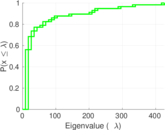### Spectral graph drawing based on the adjacency matrix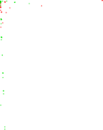### Spectral graph drawing based on the Laplacian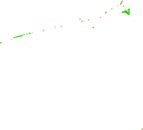### Spectral graph drawing based on the normalized adjacency matrix### Degree assortativity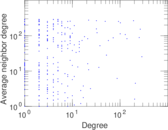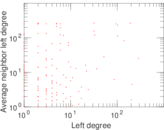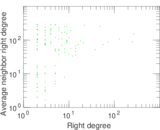### Zipf plot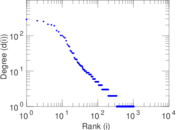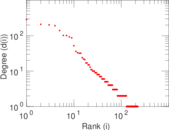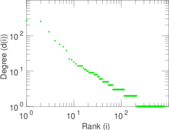### Hop distribution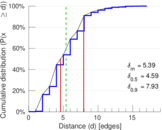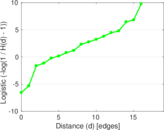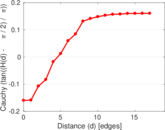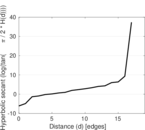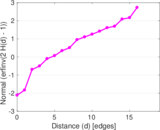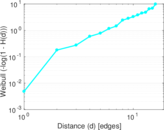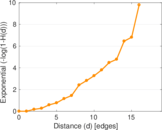### Double Laplacian graph drawing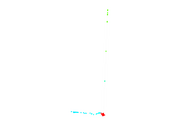### Delaunay graph drawing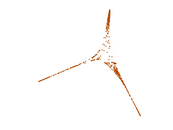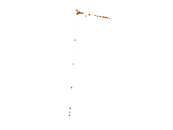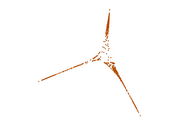### Edge weight/multiplicity distribution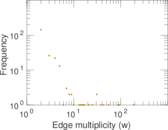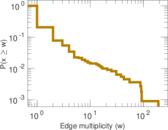### Temporal distribution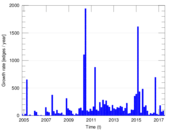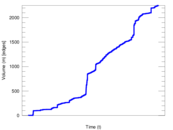### Temporal hop distribution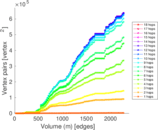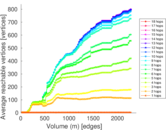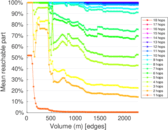### Diameter/density evolution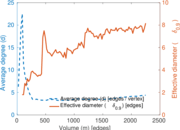### Matrix decompositions plots